# Sharing money

A certain sum of money is shared in the ratio 2 :3:9. If the difference between the first and the second is $40, then the amount of money shared is ### Correct answer: x = 560 ### Step-by-step explanation: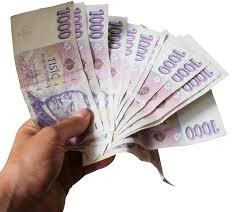Did you find an error or inaccuracy? Feel free to write us. Thank you!Tips to related online calculators Need help to calculate sum, simplify or multiply fractions? Try our fraction calculator. Check out our ratio calculator. Do you have a linear equation or system of equations and looking for its solution? Or do you have a quadratic equation? #### You need to know the following knowledge to solve this word math problem: ## Related math problems and questions: • Exchange ratesIf the Canadian dollar appreciated by C$0.005 relative to the US dollar, what would be the new value of the Canadian dollar per US dollar? Assume the current exchange rate was US$1 = C$0.907.
• LoanIf you take a bank loan $10000 and we want to repay after the year, we have to pay the total amount$ 10320/ What is the annual interest rate on this loan?
• Saving 9An amount of $2000 is invested at an interest of 5% per month. If$ 200 is added at the beginning of each successive month but no withdrawals. Give an expression for the value accumulated after n months. After how many months will the amount has accumula
• Tyreek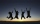Tyreek, Antuan and Alex are given a total of $3500 to be shared among themselves in the ratio 3:5:12. How much each get money? • Four pupilsFour pupils divided$ 1485 so that the second received 50% less than the first, the third 1/2 less than a fourth, and fourth $154 less than the first. How much money had each of them? • Banknotes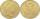$ 1390 was collected. How much was in $20 notes and how many in$50 notes in that order? How many solutions exists?
• Earnings per share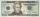The table below shows the stock price, earnings per share, and dividends per share for three companies for a recent year: Deere &Co. Price $65.70 earnings per share$4.40 Dividends per share $1.16 Google price$528.33 earnings per share $27.72 Dividends p • Sales offIf a sweater sells for$ 19 after a 5% markdown, what was its original price?
• Four friendsFour friends shared the money. Vasek got 1/4 of the total amount. Tonda received 1/3 of the rest of the money, Joe got a half from the second residue and Jirka left 80. How much money get together?
• A loanA loan in the amount of $944 is charged simple interest at an annual rate of 8.1%. How much money is owed 14 months after the loan was made? • Trio ratioHans, Alena, and Thomas have a total of 740 USD. Hans and Alena split in the ratio 5: 6 and Alena and Thomas in the ratio 4: 5. How much will everyone get? • Salary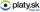Calculate the equivalent annual salary to an hourly wage of$ 19. Assume a 42-hour workweek.
• Percentages 5$5.25 is 7 1/2% of what number? • A fishermanA fisherman buys carnivores to fish. He could buy either 6 larvae and 4 worms for$ 132 or 4 larvae and 7 worms per $127. What is the price of larvae and worms? Argue the answer. • Expression money per time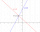You started this year with$196 saved and you continue to save an additional $19 per month. Write an algebraic expression to represent the total amount of money saved after m months. • Stacks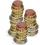Annie has a total of$ 702. The money must be divided into stacks so that each buyer has the same amount of dollars. How many options she has?
• Interest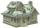What is the annual interest rate on your account if we put $x and after$n days received \$y?